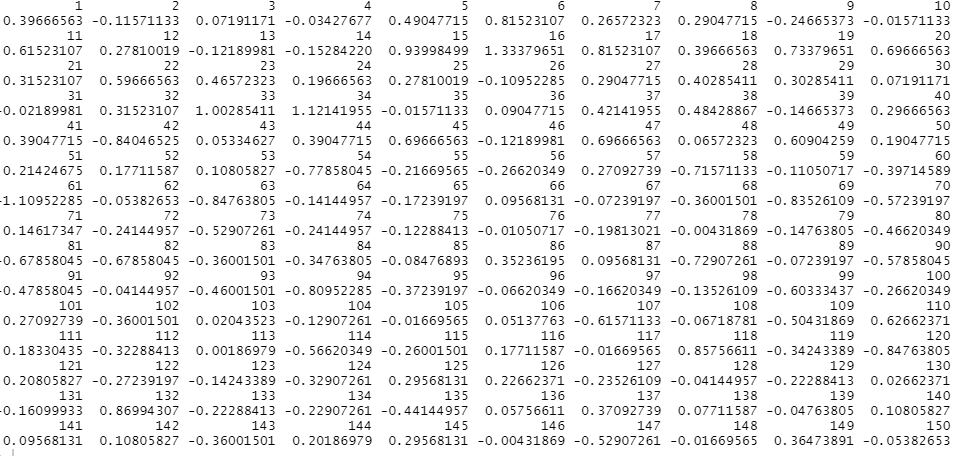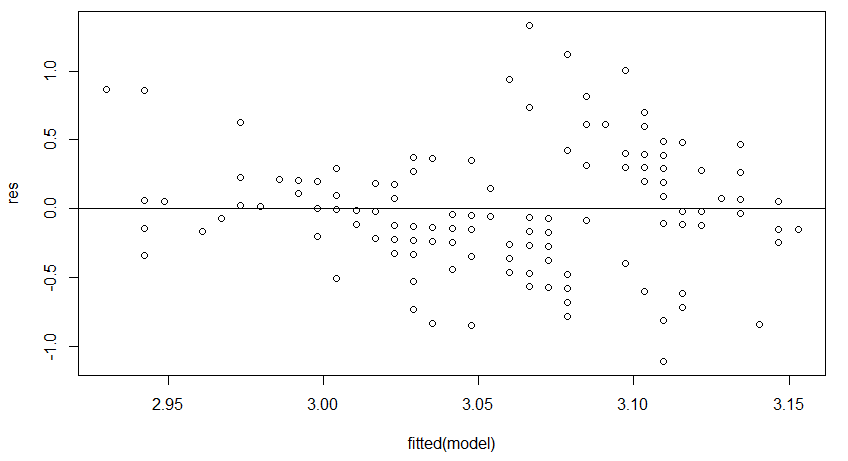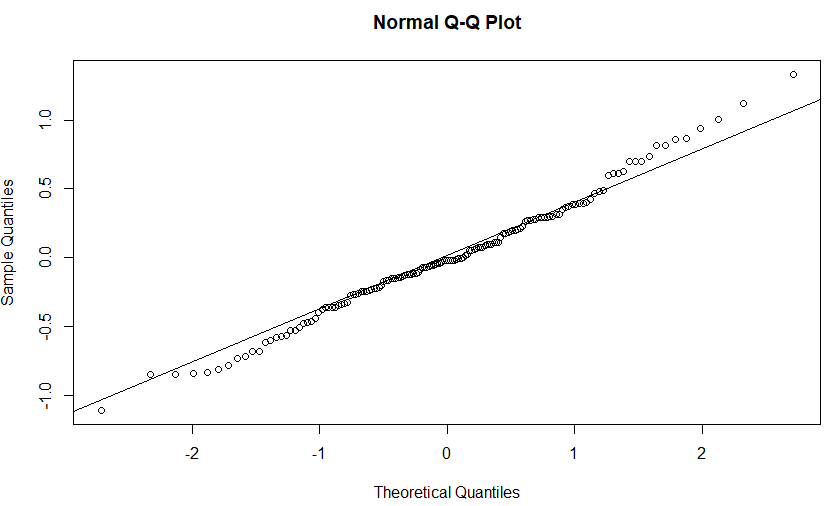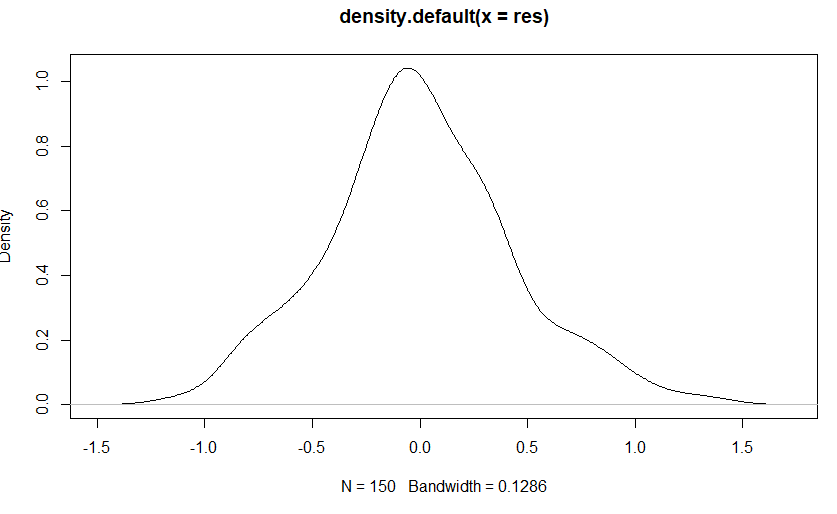# How to Create a Residual Plot in R

• Last Updated : 02 Jun, 2022

In this article, we will be looking at a step-wise procedure to create a residual plot in the R programming language.

Residual plots are often used to assess whether or not the residuals in regression analysis are normally distributed and whether or not they exhibit heteroscedasticity.

Let’s create a residual plot in R programming language.

### Step 1: Fit regression model

Under this step, we will fit a regression model using the iris data set which is the in-built dataset from the rstudio as the response variable and Sepal.Length and Sepal.Width as explanatory variables using the lm()) function and then further calling the resid() function passed this regression model passed to get the list of residuals of the model.

## R

 `# load the dataset ` `data``(``"iris"``) ` ` `  `# select Target attribute and  ` `# Predictor attribute ` `Y<- iris[,``"Sepal.Width"``]  ` `X<- iris[,``"Sepal.Length"``] ` ` `  `# fit a regression model ` `model <- ``lm``(Y~X) ` ` `  `# get list of residuals  ` `res <- ``resid``(model) ` `res `

Output:### Step 2: Produce residual vs. fitted plot

In this step, we are plotting a scatter plot of the residual of the modal vs filtered model to visually detect heteroscedasticity – e.g. a systematic change in the spread of residuals over a range of values.

## R

 `# produce residual vs. fitted plot ` `plot``(``fitted``(model), res) ` ` `  `# add a horizontal line at 0  ` `abline``(0,0) `

Output:### Step 3: Produce a Q-Q plot

Here, we are plotting a Q-Q plot using the qqnorm() function, for determining if the residuals follow a normal distribution. If the data values in the plot fall along a roughly straight line at a 45-degree angle using the qqline() function passed with the required parameters, then the data is normally distributed. And in the output, the residuals tend to stray from the line quite a bit near the tails, which indicates that they’re not normally distributed.

## R

 `# create Q-Q plot for residuals ` `qqnorm``(res) ` ` `  `# add a straight diagonal line  ` `# to the plot ` `qqline``(res)`

Output:### Step 4: Produce a density plot

In this step, we plot density plots to visually check whether or not the residuals are normally distributed. If the plot is roughly bell-shaped, then the residuals likely follow a normal distribution and as compared with the output the density plot roughly follows a bell shape, which ensures that the residuals are more normally distributed.

## R

 `plot``(``density``(res))`

Output:My Personal Notes arrow_drop_up
Recommended Articles
Page :